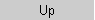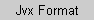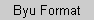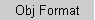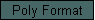## Polymake 1.3 File Format

A polytope in the polymake system is represented by a printable ASCII file. Each of its properties is represented by a sequence of non-empty lines, called a section. The first line of a section is called its title.

Lines starting with `#` are treated as comments. They can be arbitrarily intermixed with the data lines. However, procession by polymake moves all comment lines above the title of the respective section.

The title of a section contains its name optionally followed by the attribute list enclosed in parentheses. Several attributes in the list are separated by `+`. Both the name and the attributes are built only of alphanumeric characters (letters, digits, underscore). By convention, data section names are written in upper case.

### Attributes

As already mentioned, a section is defined merely as a collection of lines of ASCII characters, so that it's generally possible to introduce arbitrary sections with arbitrary contents. However, the programs comprising the polymake package need to be able to parse the data in some reasonable way, so the standard sections are expected to obey stricter syntactic rules.

While most sections contain data of a fixed type, there are certain sections which may contain data of various types. In order to distinguish between these types the following attributes are used:

 empty arbitrary precision rational data, homogeneous coordinate model `float` floating point data `dehomogenized` affine coordinate model `true`, `false` indicates a boolean type `labeled` A graph having additional data on the edges. Edge label follows the number of the adjacent node.

### Section types

The notion of a section type is introduced here for the ease of description. Polymake does not really support types. It can check the contents of the sections line by line using regular expressions, but does not take care beyond the assertion of syntactical integrity.

 boolean Section with empty contents. It always has an attribute, either `true` or `false`. cardinal Section contains a single line with one non-negative integer number. It does not have any attributes. number Section contains a single line with one rational or floating-point number. The exact type is specified by the attribute: either empty or `float`. vector Section contains a single line with a sequence of rational or floating-point numbers. The exact type is specified by the attribute: either empty or `float`. matrix Section contains at least one line vector. All vectors have the same length. The exact type is specified by the attribute: either empty or `float`. list Section contains a strictly increasing list of cardinals. The entire line is enclosed in braces. list array Section contains at least one list. The length of the lists may vary from row to row. name list Section contains alphanumeric words, one identifier per line.

Some of the sections described below are marked by `*'. This means that there are no rules which produce them. Typically, these sections are provided by the user or generated by clients.

### Basic sections

 Section name Type Description `POINTS` * matrix Points such that the polytope is their convex hull. Redundancies are allowed (i.e. double or interior points). Syntax: a line "x0 x1 .. xd" represents a point in (d+1)-space (homogeneous coordinates). Affine points are identified by x0 > 0. Points with x0 == 0 can be interpreted as rays. `VERTICES` matrix Vertices of the polyhedron. No redundancies allowed. Syntax as for `POINTS`. `INEQUALITIES` * matrix Inequalities that describe half-spaces such that the polyhedron is their intersection. Redundancies are allowed. Dual to `POINTS`. Syntax: a line "A0 A1 .. Ad" defines the (closed affine) half-space of points (1,x1,..,xd) such that A0 + A1*x1 + .. + Ad*xd >= 0. `FACETS` matrix Facets of the polyhedron. Dual to `VERTICES`. Syntax as for `INEQUALITIES`. `EQUATIONS`* matrix Equations that hold for all points of the polyhedron. Syntax: a line "A0 A1 .. Ad" describes the hyperplane of all points (1,x1,..,xd) such that A0 + A1*x1 + .. + Ad*xd == 0. `AFFINE_HULL` matrix or empty Dual basis of the affine hull of the polyhedron. Syntax as of `EQUATIONS`. `AMBIENT_DIM` cardinal Dimension of the space where the polyhedron lives in. `IN_EQ_DIM` cardinal Dimension of the span of `INEQUALITIES` and `EQUATIONS`. `DIM` cardinal Dimension of the affine hull of the polyhedron = dimension of the polyhedron. `BOUNDED` boolean Attribute: `true` if the polyhedron is a bounded polytope, `false` otherwise. `CENTERED` boolean Attribute: `true` if (1,0,0,..) is a relative interior point, `false` otherwise. This is dual to `BOUNDED`. `POSITIVE` boolean Attribute: `true` if all vertices of the polyhedron have non-negative coordinates, that is, it lies entirely in the positive orthant.

### Combinatorics

 `N_VERTICES` cardinal Number of vertices. `N_EDGES` cardinal Number of edges. `N_RIDGES` cardinal Number of ridges. `N_FACETS` cardinal Number of facets. `F_VECTOR` list fk is the number of k-faces. `H_VECTOR` list Simplicial h-vector. Defined for simplicial polytopes and also for their duals. `F2_VECTOR` matrix of cardinals fik is the number of pairs of incident pairs of i-faces and k-faces. A copy of the `F_VECTOR` is on the digonal of this symmetric matrix. `FACETS_THRU_VERTICES` list array For each vertex there is a line that contains those facets which are incident with the vertex in question. The facets are numbered from 0 to `N_FACETS-1` according to their order in the `FACETS` section. The numbers of the facets are strictly increasing. `VERTICES_IN_FACETS` list array Dual to `FACETS_THRU_VERTICES`. `GRAPH` list array For each vertex there is a line that contains the adjacent vertices. The numbers of the vertices are strictly increasing. `DUAL_GRAPH` list array Dual to `GRAPH`. `VERTEX_DEGREES` vector of cardinals For each vertex there is an entry denoting the degree of the vertex in the `GRAPH`. `FACET_DEGREES` vector of cardinals For each facet there is an entry denoting the degree of the facet in the `DUAL_GRAPH`. `VERTEX_SIZES` vector of cardinals For each vertex there is an entry denoting how many facets pass through this particular vertex. `FACET_SIZES` vector of cardinals For each facet there is an entry denoting how many vertices are contained in this particular facet. `DIAMETER` cardinal Graph theoretical diameter of `GRAPH`. `DUAL_DIAMETER` cardinal Graph theoretical diameter of `DUAL_GRAPH`. `TRIANGLE_FREE` boolean `true` if `GRAPH` does not contain a triangle, `false` otherwise. `DUAL_TRIANGLE_FREE` boolean `true` if `DUAL_GRAPH` does not contain a triangle, `false` otherwise. `ALTSHULER_DET` cardinal Let I be the vertex-facet incidence matrix, then the Altshulter determinant is defined as max{det(I*Itr),det(Itr*I)}. `SIMPLICIAL` boolean Attribute: `true` if the polytope is simplicial, `false` otherwise. `SIMPLE`, `NEIGHBORLY`, `BALANCED` boolean Similar to the above. This might be a notion that is less standard: a polytope is balanced if its dual is neighborly. `SIMPLICIALITY` cardinal Maximal dimension in which all faces are simplices. `SIMPLICITY`, `NEIGHBORLINESS`, `BALANCE` cardinal Similar to the above. `FACE_SIMPLICITY` cardinal The face simplicity is the maximal dimension in which all faces are simple polytopes. `CUBICALITY` cardinal Maximal dimension in which all faces are cubes. `CUBICAL` boolean Attribute: `true` if all facets are cubes, `false` otherwise.

For systematic reasons it would be appropriate to repeat the `DIM` section here, because it is possible to deduce the dimension of a minimal embedding space from the combinatorial structure.

### Polarization

 `VERTEX_BARYCENTER` vector The center of gravity of the vertices of a bounded polytope. `REL_INT_POINT` vector Relatively interior point of the polyhedron. `FAR_HYPERPLANE` vector Valid inequality for all affine points of the polyhedron. The corresponding hyperplane does not contain any of the points. `REVERSE_TRANSFORMATION` matrix Some invertible linear transformation that can be used to get back a previous coordinate repersentation of the polytope. It operates from the right on point row vectors; its inverse operates from the left on hyperplane column vectors.

### Optimization

 `LINEAR_OBJECTIVE` * vector Linear objective function. `ABSTRACT_OBJECTIVE` vector Abstract objective function. A vector AOF with `N_VERTICES` entries. AOF[i] is the value of the abstract objective function at vertex number i. Only defined for bounded polytopes. `MINIMAL_VALUE` number The minimal value with respect to the `ABSTRACT_OBJECTIVE` function. If the abstract linear program is unbounded, then this section is empty. `MAXIMAL_VALUE` number Similar to `MINIMAL_VALUE`. `MINIMAL_FACE` list Contains the list of vertices at which the minimum of the `ABSTRACT_OBJECTIVE` function is attained. `MAXIMAL_FACE` list Similar to `MINIMAL_FACE`. `DIRECTED_GRAPH` list array For each vertex there is a line that contains the adjacent vertices which are better with respect to the specified `ABSTRACT_OBJECTIVE` function. The numbers of the vertices are strictly increasing. `VARIABLE_NAMES` * name list You can give a list of variable names here (this amounts to naming the columns of the coordinate vectors for `VERTICES`). This is of not much use for polymake. But this information will be used by programs which convert polymake format to other formats (such as LP).

### Triangulation and volume

Everything in this section is defined for bounded polytopes only.

 `TRIANGULATION` list array Some triangulation of the polytope without interior points. `TRIANGULATION_INT` list array Some triangulation of the polytope with interior points from the `POINTS` section. `VOLUME` number Volume of the polytope.

### Lattice points

 `N_NON_NEG_INT` cardinal Number of non-negative interior lattice points.

### Oriented matroids

 `CHIROTOPE` Sequence of `+`, `-`, `0`. Chirotope corresponding to the `VERTICES`. `CHIROTOPE_INT` Sequence of `+`, `-`, `0`. Chirotope corresponding to the `POINTS`. That is, interior points are taken into account.

### Visualization and Related Stuff

 `SCHLEGEL_FACET` cardinal Number of the facet which is the Schlegel diagram is projected on. The default is 0, corresponding to the first facet listed in the section `FACETS`. Run the program `schlegel` directly for other projections. `SCHLEGEL_VERTICES` matrix Coordinates in affine 3-space of the vertices which correspond to a 3-dimensional (Schlegel-) projection of a 4-polytope. The facet which is projected on is specified in `SCHLEGEL_FACET`. `GALE_TRANSFORM` matrix Coordinates of the Gale transform. `VERTEX_COLORS` matrix Each row contains RGB-values for the corresponding vertex. This section is read by the graphlet interface. It is primarily meant to display height with respect to a linear objective function.

### Even More Sections

There might be other sections contained in a polymake file. Some of them will correspond to extensions of individual users. Others are used by the polymake system for internal use. By now this is only the `DEPENDENCES` section.